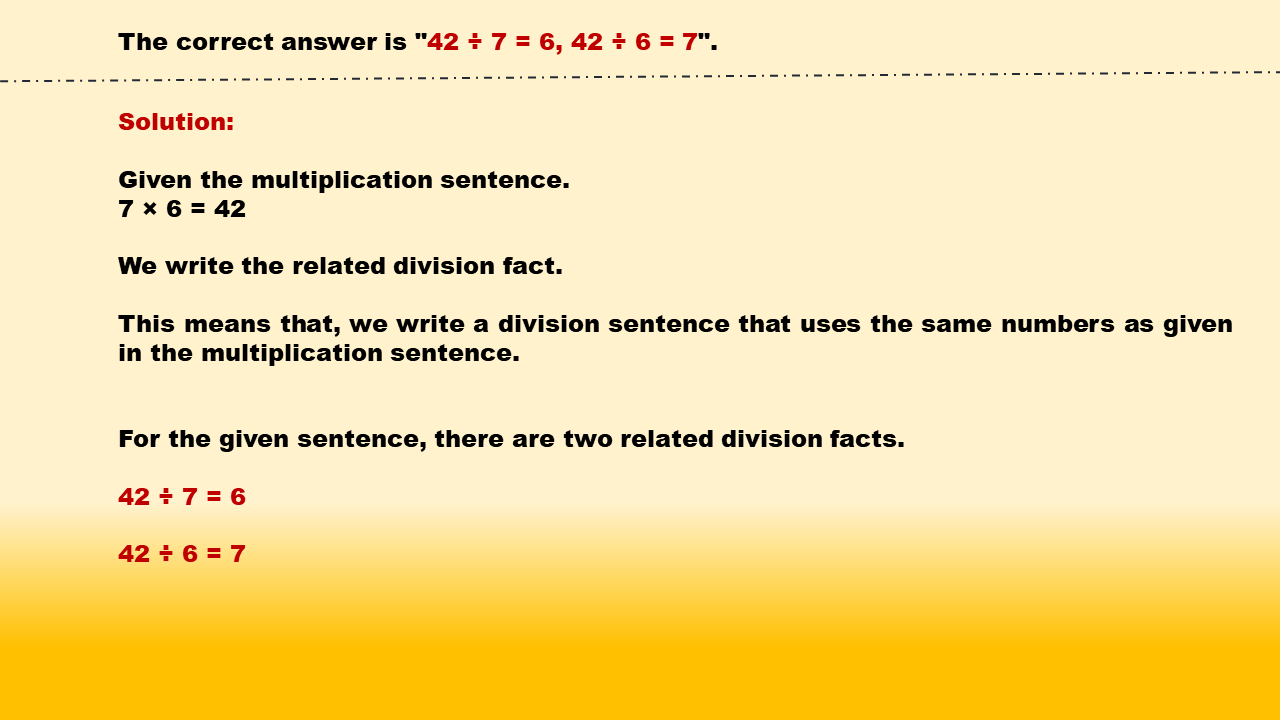1
visibility

Identify related division facts for 7 × 6 = 42.

• 48 ÷ 8 = 6, 48 ÷ 6 = 8

• 70 ÷ 7 = 10, 70 ÷ 10 = 7

• 42 ÷ 7 = 6, 42 ÷ 6 = 7

• 24 ÷ 8 = 3, 24 ÷ 3 = 8